# Calculate the difference between two dates (days/months)Calculating the difference between two dates is a common requirement and one that you will likely need to do at some point. Below you will find the best practice for calculating the difference between two days, and the best practice for calculating the difference between two months.

While there are multiple ways to get to the answer you're looking for, often the simplest solution is the best. When calculating the difference between two dates (days or months), use this approach first:

For date formatted line items, use: line item 1 – line item 2.

For time formatted line items, use: START(line item 1) – START(line item 2).

## Dates

1. Simple dates difference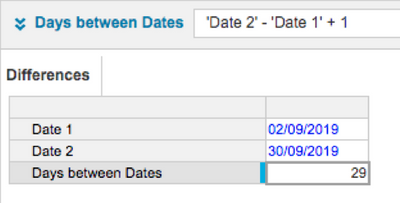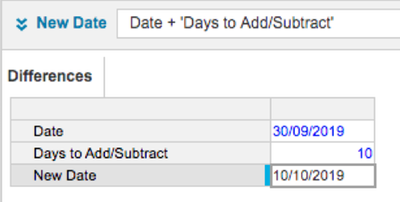3. Dates subtraction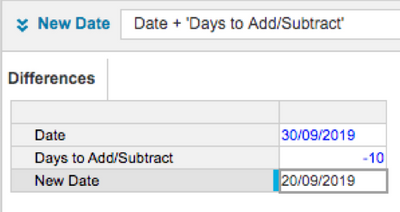## Time periods

1. Time period difference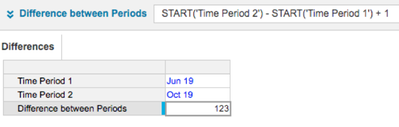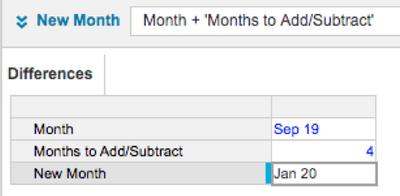3. Months subtraction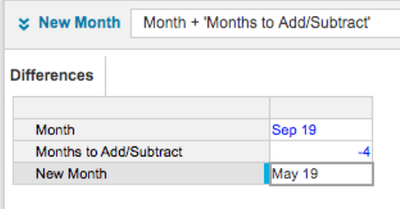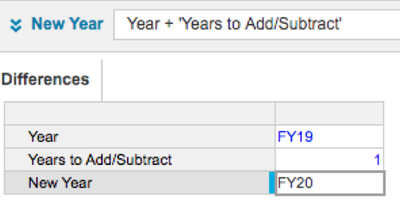5. Years subtraction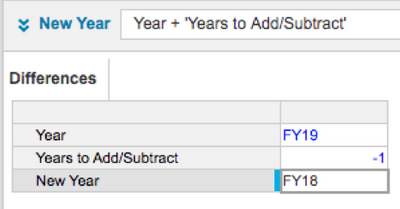## Keep 'superset' in mind when selecting your time period

If you choose a time period that is outside of the superset, it will display as blank. The superset is the earliest date from the model calendar and any time range through to the later of the last date of the calendar of any time range.

Example model: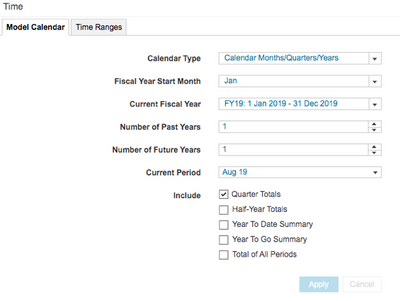Time: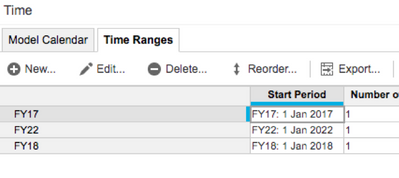Superset = Jan 17 - Dec 22: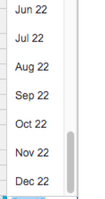If you go outside of that range: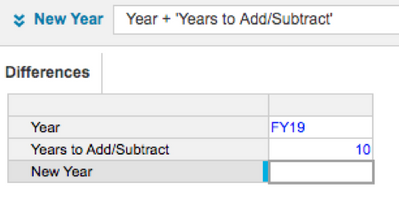Tagged:

•Thank you David! Sometimes I forget how easy is to use substract and add with time.

Sometimes we also need to go outside of the defined time period and include those time ranges in our calculation.

In this case, what would you recommend to do?

•Great article, @DavidSmith  - simple and effective.

Also, does the kudos button for the article appear in a strange place for anyone else?

•@CallumW - you mean under the "labels" box? Weird!

@emilydunn - worth having a look at why "kudos" doesn't appear directly under the article but off to the side or is it meant to be like that?•@andrewtye- precisely!

I've noticed kudos buttons dotted around in weird areas sometimes, but this one looked particularly odd!

•@andrewtye You are correct about kudos.  In a future update we'll be resolving this so that it is in the same spot for both Blogs and Knowledge Base articles.

•Great post David. One workaround I've used to find the difference in months between 2 time periods (to @Mitch_Max point)  is creating a sequential order against the time dimension using the formula below, and then just leveraging that in calculations through out the model.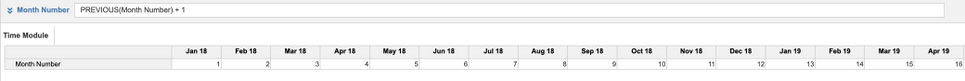Then once that is in place you can look up the difference between the periods using a simple lookup. This can be replicated at the week, month, quarter, year levels as well.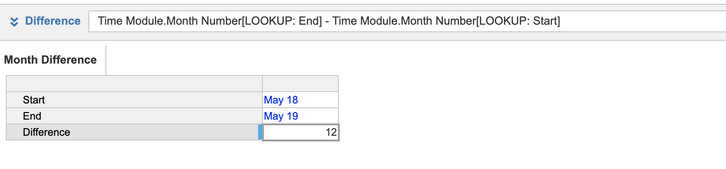•That's correct @Austinv but assumes that both periods are within the Time Range in Time Module.  This may not always be the case -  for example, when computing the length of time of a contract or relationship where the start time precedes the Time Range, or extends afterwards.

Mitch

•@DavidSmith's information is helpful but needs a clarification in the instance when you're forcasting a date outside of your model range. (I did not find the formula Mitch Max gave to give accurate months)

What worked for me:

1. Set up a time range that will cover your near future planning date needs (2010-2040)
1. You do not need to apply this time range to your line items, it just needs to exist so Anaplan recognizes your dates inbetween.
2. Set up a number-formatted line item (you can change decimals to 0 so your answer rounds) and do a simple formula: "( Budgeted Date - Reforecasted Date ) /30"

This will return the number of months between your two dates. If you want the days just get rid of the '/30' in the formula. If you want years, just change the '30' to '364' or '365'.

•@DavidSmith and @Mitch_Max, thanks for thet short guide!